# RD Sharma Solutions for Class 11 Chapter 23 - The Straight Lines Exercise 23.19

Lastly in this exercise, we shall learn the family of lines through the intersection of two given lines. Students who are looking for a valuable resource for preparing for the board exams can quickly refer to RD Sharma Class 11 Maths Solutions, which is the best reference material. It contains solutions for all the exercises of this chapter, which are prepared by expert faculty team members at BYJU’S. The solutions are created with illustrations to make concepts clear and clarify doubts instantly for students of all levels. The solutions are available in the pdf format, which can be downloaded easily from the links provided below and can be used for future reference as well.

## Download the pdf of RD Sharma Solutions for Class 11 Maths Exercise 23.19 Chapter 23 – The Straight Lines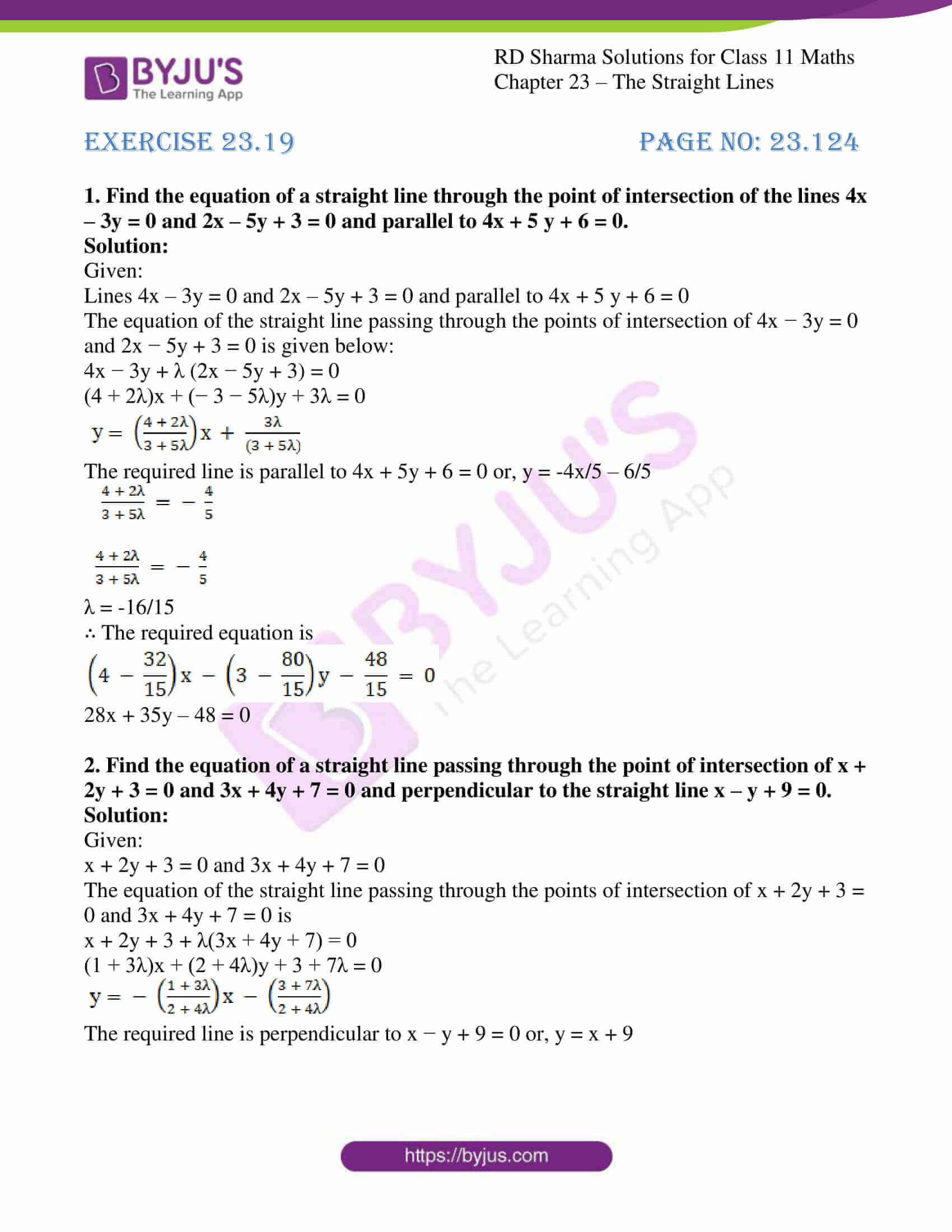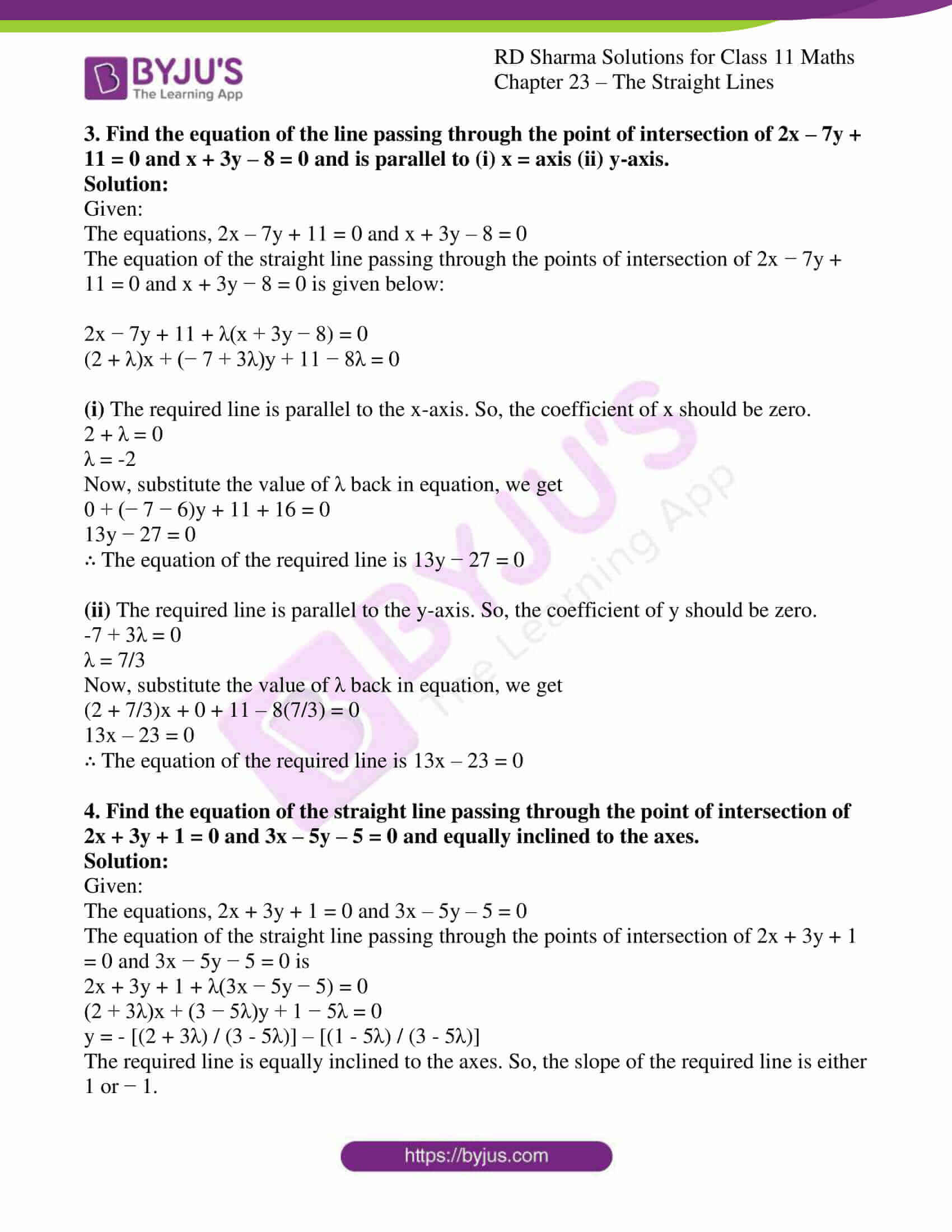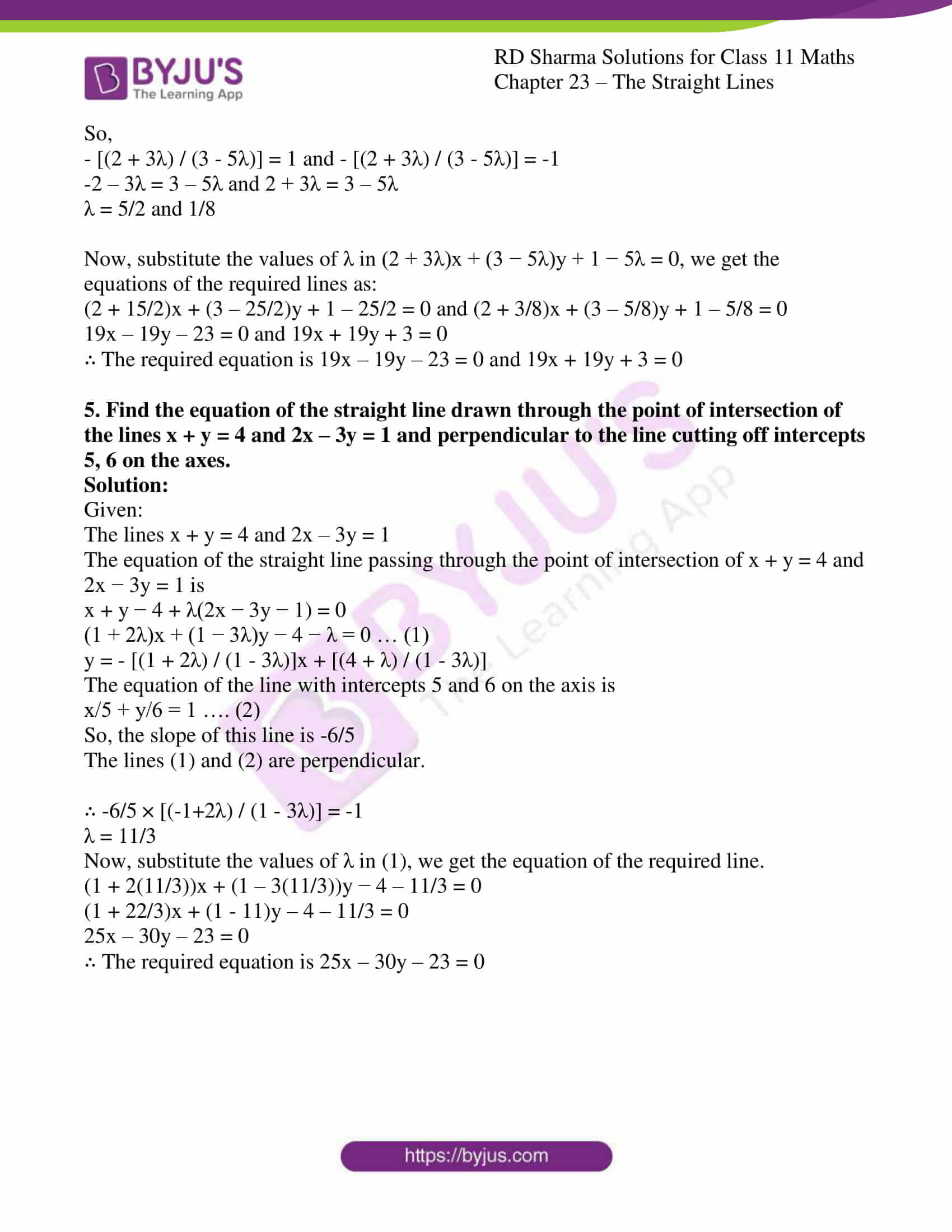### Access answers to RD Sharma Solutions for Class 11 Maths Exercise 23.19 Chapter 23 – The Straight Lines

#### EXERCISE 23.19 PAGE NO: 23.124

1. Find the equation of a straight line through the point of intersection of the lines 4x – 3y = 0 and 2x – 5y + 3 = 0 and parallel to 4x + 5 y + 6 = 0.

Solution:

Given:

Lines 4x – 3y = 0 and 2x – 5y + 3 = 0 and parallel to 4x + 5 y + 6 = 0

The equation of the straight line passing through the points of intersection of 4x − 3y = 0 and 2x − 5y + 3 = 0 is given below:

4x − 3y + λ (2x − 5y + 3) = 0

(4 + 2λ)x + (− 3 − 5λ)y + 3λ = 0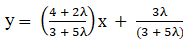The required line is parallel to 4x + 5y + 6 = 0 or, y = -4x/5 – 6/5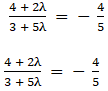λ = -16/15

∴ The required equation is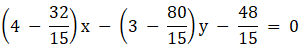28x + 35y – 48 = 0

2. Find the equation of a straight line passing through the point of intersection of x + 2y + 3 = 0 and 3x + 4y + 7 = 0 and perpendicular to the straight line x – y + 9 = 0.

Solution:

Given:

x + 2y + 3 = 0 and 3x + 4y + 7 = 0

The equation of the straight line passing through the points of intersection of x + 2y + 3 = 0 and 3x + 4y + 7 = 0 is

x + 2y + 3 + λ(3x + 4y + 7) = 0

(1 + 3λ)x + (2 + 4λ)y + 3 + 7λ = 0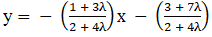The required line is perpendicular to x − y + 9 = 0 or, y = x + 9

3. Find the equation of the line passing through the point of intersection of 2x – 7y + 11 = 0 and x + 3y – 8 = 0 and is parallel to (i) x = axis (ii) y-axis.

Solution:

Given:

The equations, 2x – 7y + 11 = 0 and x + 3y – 8 = 0

The equation of the straight line passing through the points of intersection of 2x − 7y + 11 = 0 and x + 3y − 8 = 0 is given below:

2x − 7y + 11 + λ(x + 3y − 8) = 0

(2 + λ)x + (− 7 + 3λ)y + 11 − 8λ = 0

(i) The required line is parallel to the x-axis. So, the coefficient of x should be zero.

2 + λ = 0

λ = -2

Now, substitute the value of λ back in equation, we get

0 + (− 7 − 6)y + 11 + 16 = 0

13y − 27 = 0

∴ The equation of the required line is 13y − 27 = 0

(ii) The required line is parallel to the y-axis. So, the coefficient of y should be zero.

-7 + 3λ = 0

λ = 7/3

Now, substitute the value of λ back in equation, we get

(2 + 7/3)x + 0 + 11 – 8(7/3) = 0

13x – 23 = 0

∴ The equation of the required line is 13x – 23 = 0

4. Find the equation of the straight line passing through the point of intersection of 2x + 3y + 1 = 0 and 3x – 5y – 5 = 0 and equally inclined to the axes.

Solution:

Given:

The equations, 2x + 3y + 1 = 0 and 3x – 5y – 5 = 0

The equation of the straight line passing through the points of intersection of 2x + 3y + 1 = 0 and 3x − 5y − 5 = 0 is

2x + 3y + 1 + λ(3x − 5y − 5) = 0

(2 + 3λ)x + (3 − 5λ)y + 1 − 5λ = 0

y = – [(2 + 3λ) / (3 – 5λ)] – [(1 – 5λ) / (3 – 5λ)]

The required line is equally inclined to the axes. So, the slope of the required line is either 1 or − 1.

So,

– [(2 + 3λ) / (3 – 5λ)] = 1 and – [(2 + 3λ) / (3 – 5λ)] = -1

-2 – 3λ = 3 – 5λ and 2 + 3λ = 3 – 5λ

λ = 5/2 and 1/8

Now, substitute the values of λ in (2 + 3λ)x + (3 − 5λ)y + 1 − 5λ = 0, we get the equations of the required lines as:

(2 + 15/2)x + (3 – 25/2)y + 1 – 25/2 = 0 and (2 + 3/8)x + (3 – 5/8)y + 1 – 5/8 = 0

19x – 19y – 23 = 0 and 19x + 19y + 3 = 0

∴ The required equation is 19x – 19y – 23 = 0 and 19x + 19y + 3 = 0

5. Find the equation of the straight line drawn through the point of intersection of the lines x + y = 4 and 2x – 3y = 1 and perpendicular to the line cutting off intercepts 5, 6 on the axes.

Solution:

Given:

The lines x + y = 4 and 2x – 3y = 1

The equation of the straight line passing through the point of intersection of x + y = 4 and 2x − 3y = 1 is

x + y − 4 + λ(2x − 3y − 1) = 0

(1 + 2λ)x + (1 − 3λ)y − 4 − λ = 0 … (1)

y = – [(1 + 2λ) / (1 – 3λ)]x + [(4 + λ) / (1 – 3λ)]

The equation of the line with intercepts 5 and 6 on the axis is

x/5 + y/6 = 1 …. (2)

So, the slope of this line is -6/5

The lines (1) and (2) are perpendicular.

∴ -6/5 × [(-1+2λ) / (1 – 3λ)] = -1

λ = 11/3

Now, substitute the values of λ in (1), we get the equation of the required line.

(1 + 2(11/3))x + (1 – 3(11/3))y − 4 – 11/3 = 0

(1 + 22/3)x + (1 – 11)y – 4 – 11/3 = 0

25x – 30y – 23 = 0

∴ The required equation is 25x – 30y – 23 = 0